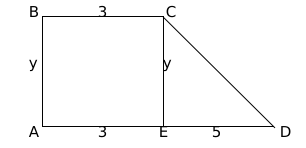Question 50

# A trapezium $$ABCD$$ has side $$AD$$ parallel to $$BC, \angle BAD = 90^\circ, BC = 3$$ cm and $$AD= 8$$ cm. If the perimeter of this trapezium is 36 cm, then its area, in sq. cm, is

SolutionCD = $$\sqrt{\ y^2+25}$$

$$11+y+\sqrt{y^2+25}=36$$

$$\sqrt{y^2+25}=25-y$$

$$y^2+25=25^2+y^2-50y$$

2y = 24

y = 12

Area of trapezium = $$3y+\frac{5y}{2}=\frac{11y}{2}=\frac{11}{2}\left(12\right)=66$$